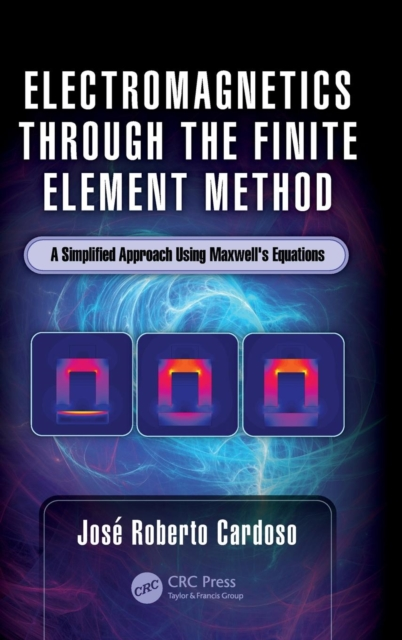# Electromagnetics through the Finite Element Method : A Simplified Approach Using Maxwell's Equations Hardback

## Edited by Jose Roberto Cardoso

Hardback

• Information

#### Description

Shelving Guide: Electrical EngineeringSince the 1980s more than 100 books on the finite element method have been published, making this numerical method the most popular.

The features of the finite element method gained worldwide popularity due to its flexibility for simulating not only any kind of physical phenomenon described by a set of differential equations, but also for the possibility of simulating non-linearity and time-dependent studies.

Although a number of high-quality books cover all subjects in engineering problems, none of them seem to make this method simpler and easier to understand. This book was written with the goal of simplifying the mathematics of the finite element method for electromagnetic students and professionals relying on the finite element method for solving design problems.

Filling a gap in existing literature that often uses complex mathematical formulas, Electromagnetics through the Finite Element Method presents a new mathematical approach based on only direct integration of Maxwell's equation.

This book makes an original, scholarly contribution to our current understanding of this important numerical method.

£130.00

£124.01